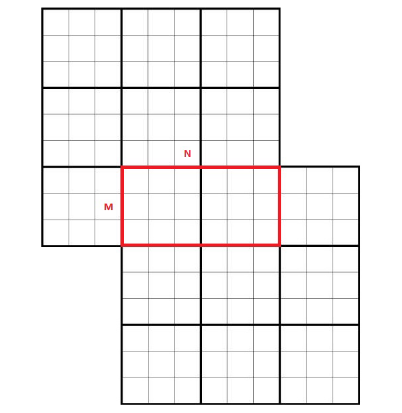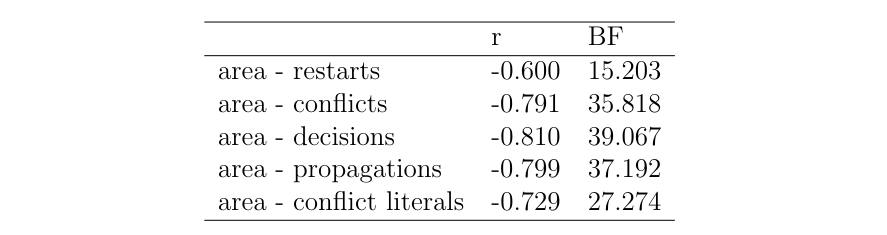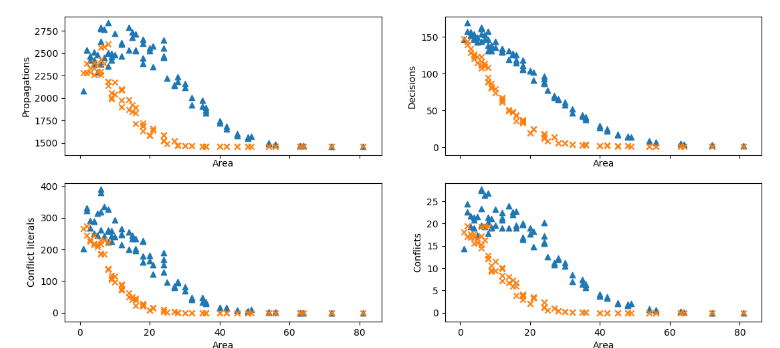# Definition and Problem Formulation

Bridge Sudokus are defined as two Sudokus having a common overlapping (bridge) region of size M x N. M and N denote the row and column overlap respectively. For a valid solution, the bridge follows the constraints of both the Sudokus.

Due to the additional constraints in the bridge Sudoku, we can expect that it is easier to solve a typical Sudoku. We note that a bridge Sudoku with no overlap is only as difficult as solving two typical Sudokus. A bridge Sudoku with a total overlap is also as ’difficult’ as solving a typical Sudoku. Therefore, as the overlap area increases, we can expect the difficulty initially increases up to a maximum and then decreases.Figure shows a “bridge” Sudoku with a M x N overlap

We want to investigate

1. If it is more difficult to solve a bridge Sudoku compared to a typical Sudoku with the same givens (givens are the numbers a Sudoku is initialized with)?
2. Does the difficulty versus overlap area plot follow an inverted parabolic structure?

We need a way to measure the ‘difficulty’ness of solving a Sudoku. For this, we use the number of conflicts, propagations, conflict literals and decisions made to solve a Sudoku using a minisat solver .

# SAT Encoding for the ‘bridge’ Sudoku

Our encoding is based on the minimal encoding strategy introduced by . This encoding technique suffices to characterize the bridge Sudoku and compare its difficulty against solving a typical Sudoku. Encoding a bridge Sudoku puzzle requires `9 ∗ 9 ∗ 9 ∗ 2 = 1458` variables. We denote the variable sₓᵧₚ as True if and only if the number z is assigned to the row x and the column y of the Sudoku p, `p ∈ {1, 2}`. To solve a typical Sudoku, the constraints are:

The block constraint is given by:

In addition to the constraints required for solving a typical Sudoku, we have another constraint for the elements in the bridge area. The variables in the bridge area of the first Sudoku must be equal to the variables in bridge area of the second Sudoku:

where subscripts 1 & 2 denote two Sudokus in a bridge Sudoku. Using the above minimal encoding, the conjunctive normal (CNF) formula will have a total of `(8829 ∗ 2 + 9 ∗ m ∗ n)` clauses. Of these, `162` are nine-ary clauses, `17496` are binary clauses and `(9∗m∗n)` are four-ary clauses. The four-ary clauses result from the bridge constraint.

# Datasets and SAT solver

The dataset used for testing contains about `80000` bridge sudokus with different overlap areas. On an average there are `52` total givens. For generating the various statistics we have used the minisat solver, which is based on the two literal watch-scheme .

Minisat gives the following statistics — number of conflicts, restarts, decisions, propagations, conflict literals, cpu time and memory used. We do not use the cpu time as a statistic to explain difficulty since the application is not sandboxed. On performing a Bayesian Correlation test, we also found that cpu time is not correlated to the overlap area and the difficulty in solving a bridge Sudoku.

In order to compare the bridge Sudoku statistics with standard Sudokus’, we used the same dataset without the bridge constraint. This is equivalent to solving the two Sudokus separately.

# Results

We observed that for the difficulty comparison problem, similar results were obtained when using minimal encoding and extended encoding. All the results reported below are for minimal encoding. The extended encoding results can be found in the above mentioned link.

We performed a Bayesian Correlation Test using JASP on the various statistics (decisions, restarts, conflicts, propagations and conflict literals) with the overlap area. For all these statistics, we found a high negative correlation with the overlap area.Table shows the Pearson correlation coefficient and the log of Bayes factor in favor of the alternative hypothesis for the various statistics considered with the overlap area

For the correlation test, we assume the pairs of variables follow a bivariate normal distribution. We define the null hypothesis that the population Pearson product-moment correlation between the pairs of variables equals 0. The Bayes factor values in the Table show a strong evidence for the alternate hypothesis which represents a high correlation. An interesting result that we observed was the high correlation between the number of conflicts and propagations (Pearson’s coefficient, `r = 0.993`). We generated the above mentioned statistics for the standard sudokus using the same datasets (without the bridge constraint). On average, the total number of givens were about `21%` of the total area. For each overlap area value, we generated the statistics for about a thousand different sudokus and took the average for each of the mentioned statistics.

In general, it is easier to solve bridge Sudoku compared to a typical Sudoku.Number of conflicts, propagations, conflict literals and decisions plotted against overlap area. Orange points belong to the set of bridge sudokus and the blue points belong to the corresponding standard sudokus. Note that there are lesser conflicts while solving the bridge Sudokus.

We believe that due to the additional constraints imposed on the bridge Sudoku results in fewer conflicts. Assignment of a value to one of the variables in the overlap area in effect reduces more number of clauses in the case of bridge sudokus. In addition, the solution space is more constrained.

# References

1. N. Sorensson, N. Een, Minisat v1.13 — A SAT solver with Conflict-Clause Minimization (2005).
2. I. Lynce, J. Ouaknine, Sudoku as a SAT Problem, ISAIM (2006).

--

--